Largest prime power divisor

This article defines an arithmetic function or number-theoretic function: a function from the natural numbers to a ring (usually, the ring of integers, rational numbers, real numbers, or complex numbers).
View a complete list of arithmetic functions

Definition

Letbe a natural number. The largest prime power divisor of, sometimes denoted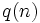and sometimes denoted, is defined as the largest prime power that divides.

Behavior

The ID of the sequence in the Online Encyclopedia of Integer Sequences is A034699

Upper bound

The value of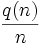is largest whenitself is a prime power, namely, it isfor these values of. Since there are infinitely many primes, we have:.

Lower bound

Further information: Largest prime power divisor has logarithmic lower bound

The largest prime power divisor ofis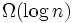. In fact, we have: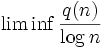is finite and greater than zero.

Thus, we have:.

Asymptotic fraction

Further information: Fractional distribution of largest prime power divisor

The value ofis almost uniformly distributed in the interval.

Relation with other arithmetic functions

• Prime divisor count function: This is the total number of prime divisors of, and is denoted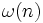. We have the following relation:.